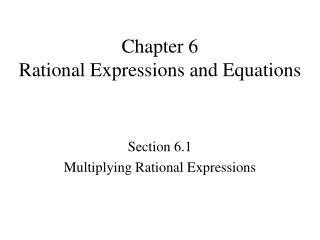DownloadDownload PresentationChapter 6 Rational Expressions and Equations

# Chapter 6 Rational Expressions and Equations

Télécharger la présentation## Chapter 6 Rational Expressions and Equations

- - - - - - - - - - - - - - - - - - - - - - - - - - - E N D - - - - - - - - - - - - - - - - - - - - - - - - - - -
##### Presentation Transcript

1. Chapter 6Rational Expressions and Equations Section 6.1 Multiplying Rational Expressions

2. HW #6.1Pg 248 1-37Odd, 40-43

3. Chapter 6Rational Expressions and Equations Section 6.2 Addition and Subtraction

4. 9. 10. 11. 12. 13. 14. 16. 15. 13. 12. 11. 10. 9. 14. 16. 15.

5. LOGICAL REASONING Tell whether the statement is always true, sometimes true, or never true. Explain your reasoning. • The LCD of two rational expressions is the product of the denominators. • Sometimes • The LCD of two rational expressions will have a degree greater than or equal to that of the denominator with the higher degree. • Always

6. 17. 18. 19. Simplify the expression.

7. 20.

8. HW #6.2 Pg 253-254 3-30 Every Third Problem 31-45 Odd

9. Chapter 6Rational Expressions and Equations 6.3 Complex Rational Expressions

10. HW 6.3Pg 258 1-23 Odd, 26-28

11. HW Quiz 6.3Wednesday, March 12, 2014

12. Chapter 6Rational Expressions and Equations 6.4 Division of Polynomials

13. Do a few examples of a poly divided by a monomial • Discuss the proof of the remainder theorem

14. HW #6.4Pg 262 1-25 Odd, 26-32

15. Chapter 6Rational Expressions and Equations Section 6.5 Synthetic Division

16. Part 1 Dividing using Synthetic Division Objective: Use synthetic division to find the quotient of certain polynomials • Algorithm • A systematic procedure for doing certain computations. • The Division Algorithm used in section 6.4 can be shortened if the divisor is a linear polynomial • Synthetic Division

17. Part 1 Dividing using Synthetic Division EXAMPLE 1 To see how synthetic division works, we will use long division to divide the polynomial by

18. Dividing PolynomialsUsing Synthetic Division

19. Set divisor = 0 and solve. Put answer here. x + 3 = 0 so x = - 3 Bring first number down below line Multiply these and put answer above line in next column Multiply these and put answer above line in next column Multiply these and put answer above line in next column Synthetic Division There is a shortcut for long division as long as the divisor is x – k where k is some number. (Can't have any powers on x). 1 - 3 1 6 8 -2 - 3 Add these up - 9 3 Add these up Add these up 1 x2 + x 3 - 1 1 This is the remainder Put variables back in (one x was divided out in process so first number is one less power than original problem). So the answer is: List all coefficients (numbers in front of x's) and the constant along the top. If a term is missing, put in a 0.

20. Set divisor = 0 and solve. Put answer here. x - 4 = 0 so x = 4 Bring first number down below line Multiply these and put answer above line in next column Multiply these and put answer above line in next column Multiply these and put answer above line in next column Multiply these and put answer above line in next column Let's try another Synthetic Division 0 x3 0 x 1 4 1 0 - 4 0 6 4 Add these up 16 48 192 Add these up Add these up Add these up 1 x3 + x2 + x + 4 12 48 198 This is the remainder Now put variables back in (remember one x was divided out in process so first number is one less power than original problem so x3). So the answer is: List all coefficients (numbers in front of x's) and the constant along the top. Don't forget the 0's for missing terms.

21. Bring first number down below line Multiply these and put answer above line in next column Multiply these and put answer above line in next column Multiply these and put answer above line in next column Let's try a problem where we factor the polynomial completely given one of its factors. You want to divide the factor into the polynomial so set divisor = 0 and solve for first number. - 2 4 8 -25 -50 - 8 Add these up 0 50 Add these up Add these up No remainder so x + 2 IS a factor because it divided in evenly 4 x2 + x 0 - 25 0 Put variables back in (one x was divided out in process so first number is one less power than original problem). So the answer is the divisor times the quotient: List all coefficients (numbers in front of x's) and the constant along the top. If a term is missing, put in a 0. You could check this by multiplying them out and getting original polynomial

22. HW #6.5Pg 265 1-19

23. 6-6 Solving Rational Equation . . . And Why To solve problems using rational equations

24. A rational equation is an equation that contains one or more rational expressions. These are rational equations. To solve a rational equation, we multiply both sides by the LCD to clear fractions.